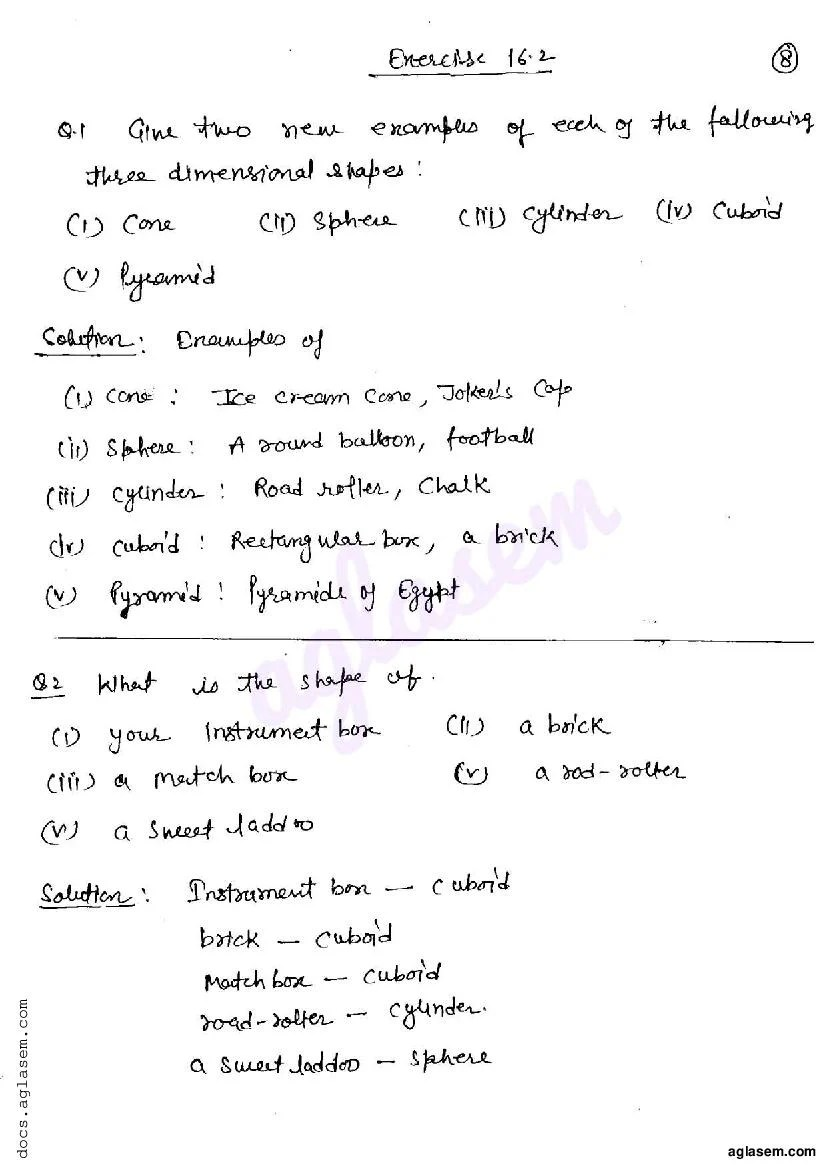# RD Sharma Solutions Class 6 Chapter 16 Understanding Three Dimensional Shapes Exercise 16.2

Here you can get free RD Sharma Solutions for Class 6 Maths Chapter 16 Understanding Three Dimensional Shapes Exercise 16.2. All RD Sharma Book Solutions are given here exercise wise for the chapter Understanding Three Dimensional Shapes. RD Sharma Solutions are helpful in the preparation of several school level, graduate and undergraduate level competitive exams. Practicing questions from RD Sharma Mathematics Solutions for Class 6 Chapter 16 Understanding Three Dimensional Shapes is proven to enhance your math skills.

 Class: Class 6th Chapter: Chapter 16 Exercise: Exercise 16.2 Name: Understanding Three Dimensional Shapes

## RD Sharma Solutions Class 6 Chapter 16 Understanding Three Dimensional Shapes Exercise 16.2

RD Sharma Class 6 Solutions Chapter 16 for Understanding Three Dimensional Shapes Exercise 16.2 are given below.

RD Sharma Solutions Class 6 Maths Chapter 16 Understanding Three Dimensional Shapes Exercise 16.2RD Sharma Solutions for Class 6 Maths PDF download for Chapter 16 Understanding Three Dimensional Shapes Exercise 16.2 is available here for free.

 « Previous Next »

### RD Sharma Solutions Class 6 Chapter 16 Exercise Wise

You can also see RD Sharma Solutions of all exercises of Chapter 16 – Understanding Three Dimensional Shapes from here.

### RD Sharma Solutions Class 6 Chapter Wise

If you want RD Sharma solutions of any topic other than Chapter 16 Understanding Three Dimensional Shapes, check it from here. Mathematics by RD Sharma has all chapters that are in your Class 6 syllabus.

Click to rate this post!
[Total: 3 Average: 5]